# Tag Archives: Simple linear regression# RANSAC Regression in Python

RANSAC is an acronym for Random Sample Consensus. What this algorithm does is fit a regression model on a subset of data that the algorithm judges as inliers while removing outliers. This naturally improves the fit of the model due to the removal of some data points.The process that is used to determine inliers and outliers is described below.

1. The algorithm randomly selects a random amount of samples to be inliers in the model.
2. All data is used to fit the model and samples that fall with a certain tolerance are relabeled as inliers.
3. Model is refitted with the new inliers
4. Error of the fitted model vs the inliers is calculated
5. Terminate or go back to step 1 if a certain criterion of iterations or performance is not met.

In this post, we will use the tips data from the pydataset module. Our goal will be to predict the tip amount using two different models.

1. Model 1 will use simple regression and will include total bill as the independent variable and tips as the dependent variable
2. Model 2 will use multiple regression and  includes several independent variables and tips as the dependent variable

The process we will use to complete this example is as follows

1. Data preparation
2. Simple Regression Model fit
3. Simple regression visualization
4. Multiple regression model fit
5. Multiple regression visualization

Below are the packages we will need for this example

```import pandas as pd
from pydataset import data
from sklearn.linear_model import RANSACRegressor
from sklearn.linear_model import LinearRegression
import numpy as np
import matplotlib.pyplot as plt
from sklearn.metrics import mean_absolute_error
from sklearn.metrics import r2_score```

Data Preparation

For the data preparation, we need to do the following

2. Create X and y dataframes
3. Convert several categorical variables to dummy variables
4. Drop the original categorical variables from the X dataframe

Below is the code for these steps

```df=data('tips')
X,y=df[['total_bill','sex','size','smoker','time']],df['tip']
male=pd.get_dummies(X['sex'])
X['male']=male['Male']
smoker=pd.get_dummies(X['smoker'])
X['smoker']=smoker['Yes']
dinner=pd.get_dummies(X['time'])
X['dinner']=dinner['Dinner']
X=X.drop(['sex','time'],1)```

Most of this is self-explanatory, we first load the tips dataset and divide the independent and dependent variables into an X and y dataframe respectively. Next, we converted the sex, smoker, and dinner variables into dummy variables, and then we dropped the original categorical variables.

We can now move to fitting the first model that uses simple regression.

Simple Regression Model

For our model, we want to use total bill to predict tip amount. All this is done in the following steps.

1. Instantiate an instance of the RANSACRegressor. We the call LinearRegression function, and we also set the residual_threshold to 2 indicate how far an example has to be away from  2 units away from the line.
2. Next we fit the model
3. We predict the values
4. We calculate the r square  the mean absolute error

Below is the code for all of this.

```ransacReg1= RANSACRegressor(LinearRegression(),residual_threshold=2,random_state=0)
ransacReg1.fit(X[['total_bill']],y)
prediction1=ransacReg1.predict(X[['total_bill']])```
```r2_score(y,prediction1)
Out: 0.4381748268686979

mean_absolute_error(y,prediction1)
Out: 0.7552429811944833```

The r-square is 44% while the MAE is 0.75.  These values are most comparative and will be looked at again when we create the multiple regression model.

The next step is to make the visualization. The code below will create a plot that shows the X and y variables and the regression. It also identifies which samples are inliers and outliers. Te coding will not be explained because of the complexity of it.

```inlier=ransacReg1.inlier_mask_
outlier=np.logical_not(inlier)
line_X=np.arange(3,51,2)
line_y=ransacReg1.predict(line_X[:,np.newaxis])
plt.scatter(X[['total_bill']][inlier],y[inlier],c='lightblue',marker='o',label='Inliers')
plt.scatter(X[['total_bill']][outlier],y[outlier],c='green',marker='s',label='Outliers')
plt.plot(line_X,line_y,color='black')
plt.xlabel('Total Bill')
plt.ylabel('Tip')
plt.legend(loc='upper left')```Plot is self-explanatory as a handful of samples were considered outliers. We will now move to creating our multiple regression model.

Multiple Regression Model Development

The steps for making the model are mostly the same. The real difference takes place in make the plot which we will discuss in a moment. Below is the code for  developing the model.

```ransacReg2= RANSACRegressor(LinearRegression(),residual_threshold=2,random_state=0)
ransacReg2.fit(X,y)
prediction2=ransacReg2.predict(X)```
```r2_score(y,prediction2)
Out: 0.4298703800652126

mean_absolute_error(y,prediction2)
Out: 0.7649733201032204```

Things have actually gotten slightly worst in terms of r-square and MAE.

For the visualization, we cannot plot directly several variables t once. Therefore, we will compare the predicted values with the actual values. The better the correlated the better our prediction is. Below is the code for the visualization

```inlier=ransacReg2.inlier_mask_
outlier=np.logical_not(inlier)
line_X=np.arange(1,8,1)
line_y=(line_X[:,np.newaxis])
plt.scatter(prediction2[inlier],y[inlier],c='lightblue',marker='o',label='Inliers')
plt.scatter(prediction2[outlier],y[outlier],c='green',marker='s',label='Outliers')
plt.plot(line_X,line_y,color='black')
plt.xlabel('Predicted Tip')
plt.ylabel('Actual Tip')
plt.legend(loc='upper left')```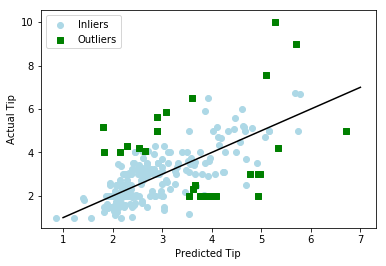The plots are mostly the same  as you cans see for yourself.

Conclusion

This post provided an example of how to use the RANSAC regressor algorithm. This algorithm will remove samples from the model based on a criterion you set. The biggest complaint about this algorithm is that it removes data from the model. Generally, we want to avoid losing data when developing models. In addition, the algorithm removes outliers objectively this is a problem because outlier removal is often subjective. Despite these flaws, RANSAC regression is another tool that can be use din machine learning.

# Using Plots for Prediction in R

It is common in machine learning to look at the training set of your data visually. This helps you to decide what to do as you begin to build your model.  In this post, we will make several different visual representations of data using datasets available in several R packages.

We are going to explore data in the “College” dataset in the “ISLR” package. If you have not done so already, you need to download the “ISLR” package along with“ggplot2” and the “caret” package.

Once these packages are installed in R you want to look at a summary of the variables use the summary function as shown below.

`summary(College)`

You should get a printout of information about 18 different variables. Based on this printout, we want to explore the relationship between graduation rate “Grad.Rate” and student to faculty ratio “S.F.Ratio”. This is the objective of this post.

Next, we need to create a training and testing dataset below is the code to do this.

```> library(ISLR);library(ggplot2);library(caret)
> data("College")
> PracticeSet<-createDataPartition(y=College\$Enroll, p=0.7, +                                  list=FALSE) > trainingSet<-College[PracticeSet,] > testSet<-College[-PracticeSet,] > dim(trainingSet); dim(testSet)
 545  18
 232  18```

The explanation behind this code was covered in predicting with caret so we will not explain it again. You just need to know that the dataset you will use for the rest of this post is called “trainingSet”.

Developing a Plot

We now want to explore the relationship between graduation rates and student to faculty ratio. We will be used the ‘ggpolt2’  package to do this. Below is the code for this followed by the plot.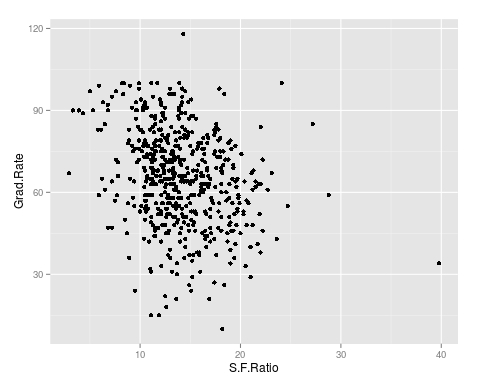As you can see, there appears to be a negative relationship between student faculty ratio and grad rate. In other words, as the ration of student to faculty increases there is a decrease in the graduation rate.

Next, we will color the plots on the graph based on whether they are a public or private university to get a better understanding of the data. Below is the code for this followed by the plot.

> qplot(S.F.Ratio, Grad.Rate, colour = Private, data=trainingSet)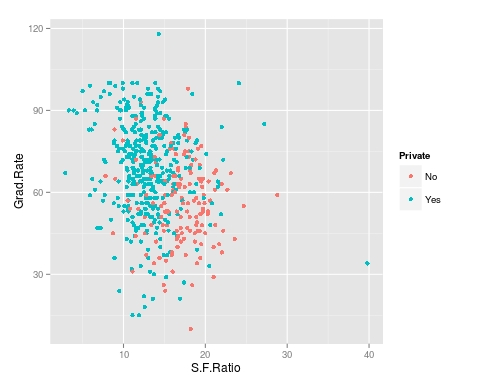It appears that private colleges usually have lower student to faculty ratios and also higher graduation rates than public colleges

We will now plot the same data but will add a regression line. This will provide us with a visual of the slope. Below is the code followed by the plot.

> collegeplot<-qplot(S.F.Ratio, Grad.Rate, colour = Private, data=trainingSet) > collegeplot+geom_smooth(method = ‘lm’,formula=y~x)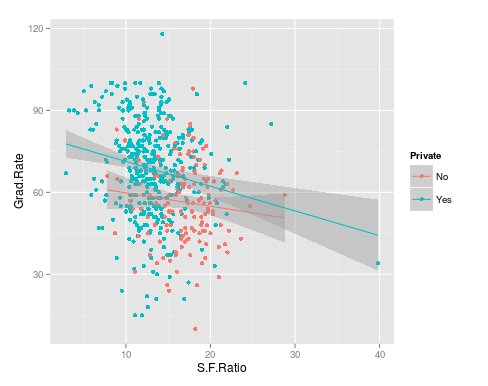Most of this code should be familiar to you. We saved the plot as the variable ‘collegeplot’. In the second line of code, we add specific coding for ‘ggplot2’ to add the regression line. ‘lm’ means linear model and formula is for creating the regression.

Cutting the Data

We will now divide the data based on the student-faculty ratio into three equal size groups to look for additional trends. To do this you need the “Hmisc” packaged. Below is the code followed by the table

```> library(Hmisc)
```
```> divide_College<-cut2(trainingSet\$S.F.Ratio, g=3)
> table(divide_College)
divide_College
[ 2.9,12.3) [12.3,15.2) [15.2,39.8]
185         179         181```

Our data is now divided into three equal sizes.

Box Plots

Lastly, we will make a box plot with our three equal size groups based on student-faculty ratio. Below is the code followed by the box plot

CollegeBP<-qplot(divide_College, Grad.Rate, data=trainingSet, fill=divide_College, geom=c(“boxplot”)) > CollegeBP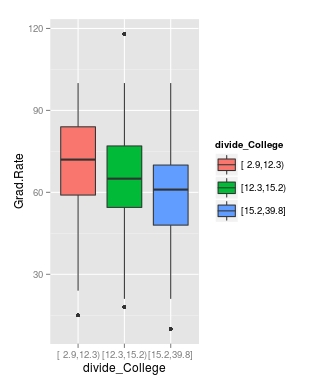As you can see, the negative relationship continues even when student-faculty is divided into three equally size groups. However, our information about private and public college is missing. To fix this we need to make a table as shown in the code below.

```> CollegeTable<-table(divide_College, trainingSet\$Private)
> CollegeTable

divide_College  No Yes
[ 2.9,12.3)  14 171
[12.3,15.2)  27 152
[15.2,39.8] 106  75```

This table tells you how many public and private colleges there based on the division of the student-faculty ratio into three groups. We can also get proportions by using the following

```> prop.table(CollegeTable, 1)

divide_College         No        Yes
[ 2.9,12.3) 0.07567568 0.92432432
[12.3,15.2) 0.15083799 0.84916201
[15.2,39.8] 0.58563536 0.41436464```

In this post, we found that there is a negative relationship between student-faculty ratio and graduation rate. We also found that private colleges have a lower student-faculty ratio and a higher graduation rate than public colleges. In other words, the status of a university as public or private moderates the relationship between student-faculty ratio and graduation rate.

You can probably tell by now that R can be a lot of fun with some basic knowledge of coding.